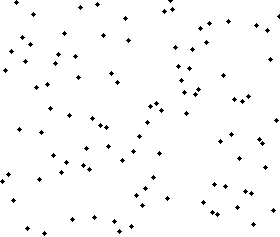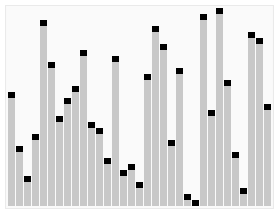# 交换排序

Wu Jun 2019-12-25 15:59:03
Categories： > Tags：

### 1 冒泡排序#### 思路

``````void BubbleSort(SqList *L)
{
int i,j;
for ( i = 1; i < L->length; i++)
{
for ( j = L->length - 1; j >= i; j--) /* 注意j是从后往前循环 */
{
if (L->r[j] > L->r[j+1]) /* 若前者大于后者（注意这里与上一算法差异) */
{
swap(L, j, j+1) /* 交换L->r[j]与L->r[j+1]的值 */
}
}
}
}
``````

#### 优化

``````void BubbleSort2(SqList* L)
{
int i,j;
Status Flag = TRUE;     /* flag用来作为标记 */
for ( i = 1; i < L->length && flag; i++) /* 若flag为true则退出循环 */
{
flag == FALSE;
for ( j = L->length - 1; j >= i; j--) /* 注意j是从后往前循环 */
{
if (L->r[j] > L->r[j+1]) /* 若前者大于后者（注意这里与上一算法差异) */
{
swap(L, j, j+1) /* 交换L->r[j]与L->r[j+1]的值 */
flag = TRUE;    /* 如果有数据交换，则flag 为 true */
}
}
}
}
``````

O(n2)

### 2 快速排序#### 思路

``````public static void quickSort(int array[],int start,int end){
if(start < end){
int n = findPoint(array,start,end);
quickSort(array,start,n-1);
quickSort(array,n+1,end);
}
}

public static int findPoint(int array[],int start,int end){
int temp = array[start];
while(start < end){
while(start < end && array[end] >= temp){
end--;
}
if(start < end){
array[start] = array[end];
}
while(start < end && array[start] <= temp){
start++;
}
if(start < end){
array[end] = array[start];
}
}
array[start] = temp;
return start;
}
``````

O(nlogn)，不稳定

### 快速排序优化

#### 1. 优化选取枢轴

``````/* 优化之一： 枢轴的选择 */
int m = low + (high - low) / 2 ; /* 计算数组中间元素的下标 */
if (L->r[low] > L->r[high])
swap(L, low, high);            /* 交换左端与右端数据，保证左端小 */
if (L->r[m] > L->r[high])
swap(L, high, m);              /* 交换中间与右端数据，保证中间小 */
if (L->r[m] > L->r[low])
swap(L, m, low);              /* 交换中间与左端数据，保证左端小 */
/* 此时L.r[low]已经为整个序列左中右三个关键数字的中间值 */
``````

#### 2. 优化不必要的交换

``````/* 优化之二：优化不必要的交换 */
int Partition1(SqList *L, int low, int high){
int pivotkey;
pivotkey = L->r[low]; /* 用于表的第一个记录作枢轴记录 */
L->r = pivotkey;   /* 将枢轴关键字备份到L->r */
while (low < high){
while (low < high && L->r[high] >= pivotkey)
high--;
L->r[low] = L->r[high]; /* 采用替换而不是交换的方式进行操作 */
while (low < high && L->r[low] <= pivotkey)
low++;
L->r[high] = L->r[low]; /* 采用替换而不是交换的方式进行操作 */
}
L->r[low] = L->r;     /* 将枢轴数值替换返回 L.r[low] */
return low; /* 返回枢轴所在位置 */
}
``````

#### 3. 优化小数组时的排序方案

``````/* 优化之三： 优化小数组时的排序方案 */
#define MAX_LENGTH_INSERT_SORT 7 /* 数组长度阈值 */
/* 对顺序表L中的子序列L.r[low...high]作快速排序 */
void QSort(SqList *L, int low, int high){
int pivot;
if (high - low > MAX_LENGTH_INSERT_SORT){
/* 当high-low大于常数时用快速排序 */
pivot = Partition(L, low, high);  /* 将L->r[low...high]一分为二，算出枢轴值pivot */
QSort(L, low, pivot - 1);         /* 对低子表递归排序 */
QSort(L, pivot + 1, high);        /* 对高子表递归排序 */
}
else /* 当high-low小于等于常数时用直接插入排序 */
InsertSort(L);
}
``````

#### 4. 优化递归操作

``````/* 优化之四: 尾递归 */
/* 对顺序表L中的子序列L-r[low...high]作快速排序 */
void QSort1(SqList *L, int low, int high)
{
int pivot;
if ((high - low) > MAX_LENGTH_INSERT_SORT)
{
while (low < high)
{
pivot = Partition(L, low, high);  /* 将L->r[low...high]一分为二，算出枢轴值pivot */
QSort(L, low, pivot - 1);         /* 对低子表递归排序 */
low = pivot + 1;        /* 尾递归 */
}
}
else
InsertSort(L);
}
``````